﻿ 基于递归神经网络的风暴潮增水预测
«上一篇文章快速检索 高级检索

 智能系统学报2017, Vol. 12Issue (5): 640-644  DOI: 10.11992/tis.2017060150

### 引用本文LEI Sen, SHI Zhenwei, SHI Tianyang, et al. Prediction of storm surge based on recurrent neural network[J]. CAAI Transactions on Intelligent Systems, 2017, 12(5): 640-644. DOI: 10.11992/tis.201706015.### 文章历史

1. 北京航空航天大学 宇航学院图像处理中心, 北京 100191;
2. 国家海洋局 北海预报中心, 山东 青岛 266000

Prediction of storm surge based on recurrent neural network
LEI Sen1, SHI Zhenwei1, SHI Tianyang1, GAO Song2, LI Yaru2, ZHONG Shan2
1. Image Processing Center, School of Astronautics, Beihang University, Beijing 100191, China;
2. Beihai Forecast Center of State Oceanic Administration, Qingdao 266000, China
Abstract: Accurately forecasting storm surges can greatly reduce personnel injuries and economic losses, and so has great practical value. Traditional methods for predicting storm surge mainly involve experience and numerical forecasting, which makes it very hard to establish accurate models. Most of today's storm surge forecast methods based on machine learning only extract the relationships among static data and fail to identify the relevant time series properties of these data. In this paper, we propose a storm surge forecast method based on the recurrent neural network. The storm surge data is rearranged with particular treatments, and an appropriate recurrent neural network is designed to perform the prediction of the time series. Compared with traditional BP neural networks, the recurrent neural network can better forecast time series data. In this study, we used a recurrent neural network to predict surges at the Weifang gauge station. The results show that the recurrent neural network produces a better prediction with a smaller error than the BP neural network.
Key words: storm surge    prediction    numerical forecast    machine learning    static data    temporal properties    BP neural networks    recurrent neural network

1 原理说明 1.1 前馈神经网络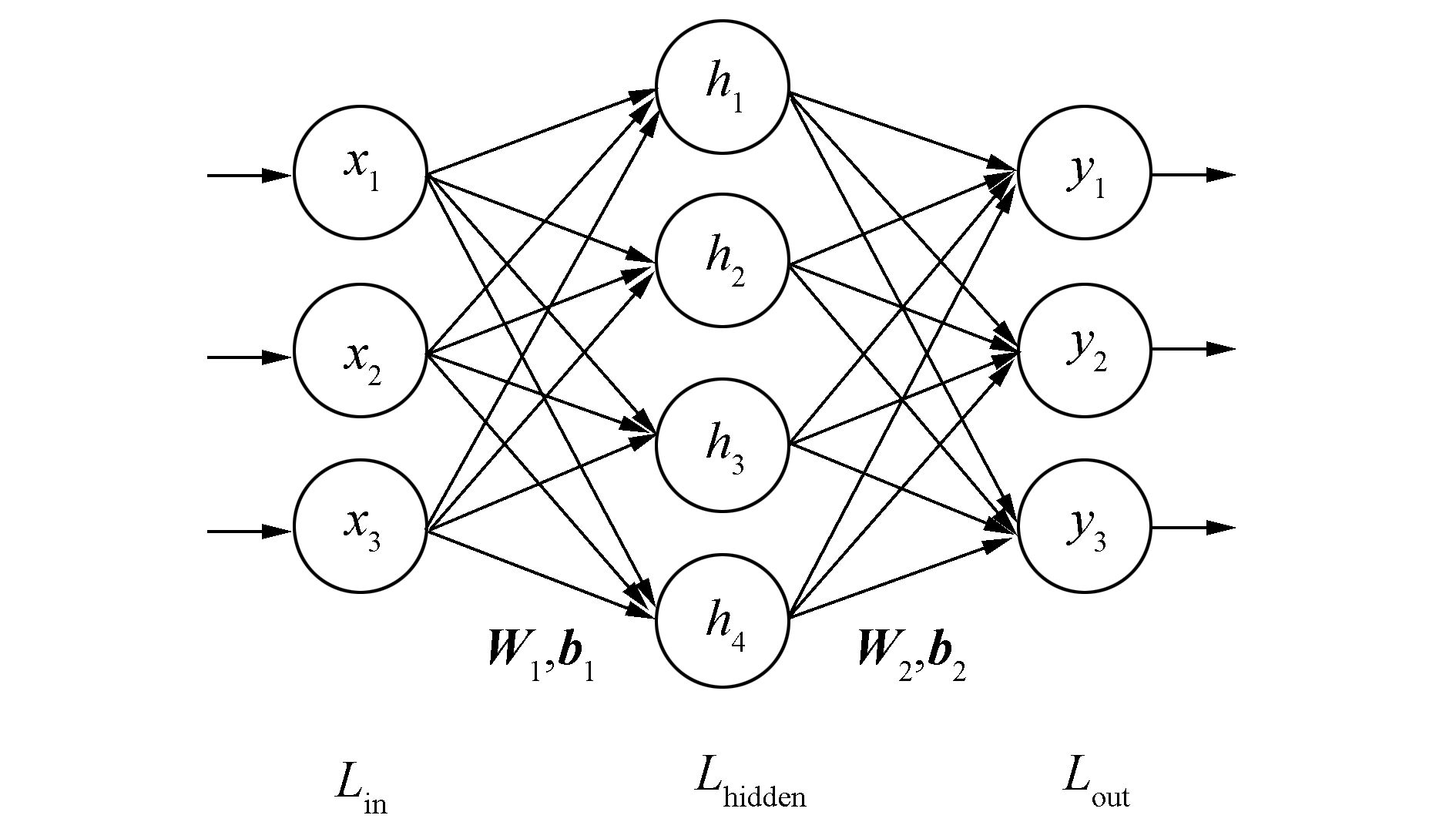图 1 多层感知器 Fig.1 Multi-layer perceptron

 $\begin{array}{l} \mathit{\boldsymbol{h}} = f\left( {\mathit{\boldsymbol{W}}_1^{\rm{T}}\mathit{\boldsymbol{x}} + {\mathit{\boldsymbol{b}}_1}} \right)\\ \mathit{\boldsymbol{y}} = f\left( {\mathit{\boldsymbol{W}}_2^{\rm{T}}\mathit{\boldsymbol{x}} + {\mathit{\boldsymbol{b}}_2}} \right) \end{array}$ (1)

1.2 递归神经网络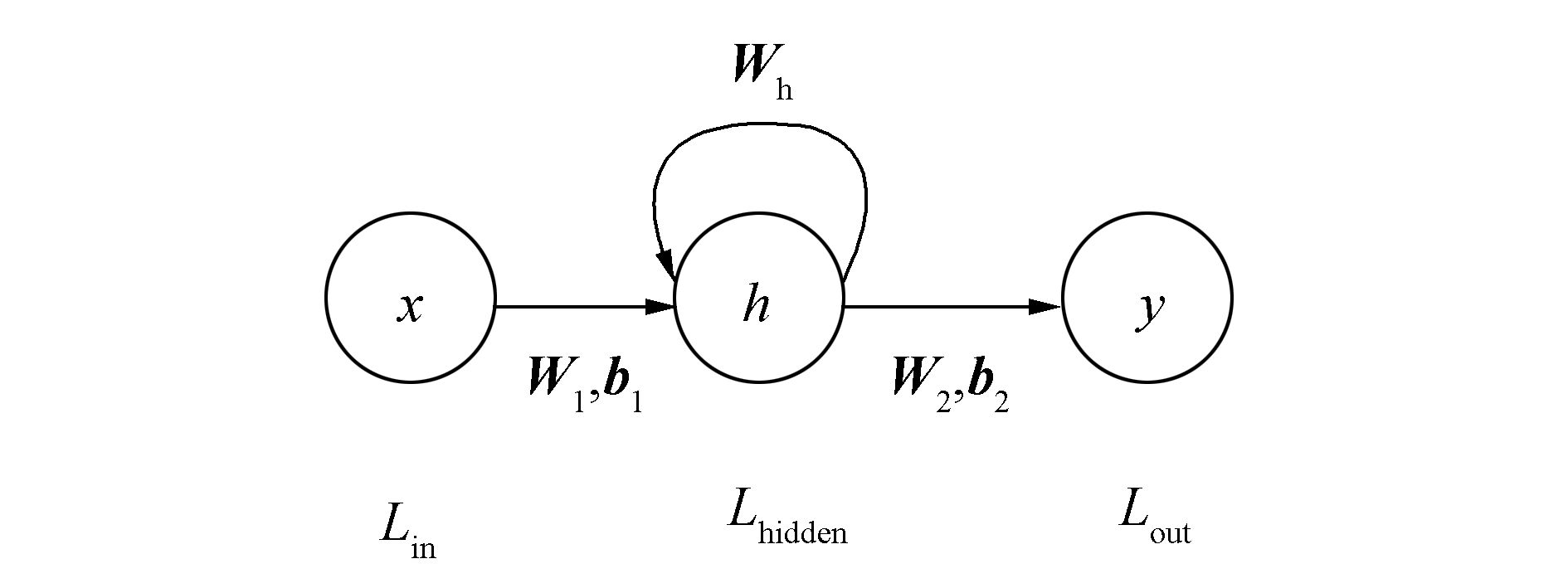图 2 递归神经网络 Fig.2 Recurrent neural networks

Lhidden隐含层包含m个神经元，则Whm×m维的权重矩阵。递归神经网络的传播过程可以用式(2)表示：

 $\begin{array}{l} {\mathit{\boldsymbol{h}}_t} = f\left( {\mathit{\boldsymbol{W}}_1^{\rm{T}}\mathit{\boldsymbol{x}} + \mathit{\boldsymbol{W}}_{\rm{h}}^{\rm{T}}{\mathit{\boldsymbol{h}}_{t - 1}} + {\mathit{\boldsymbol{b}}_1}} \right)\\ \quad \quad \mathit{\boldsymbol{y}} = f\left( {\mathit{\boldsymbol{W}}_2^{\rm{T}}{\mathit{\boldsymbol{h}}_t} + {\mathit{\boldsymbol{b}}_2}} \right) \end{array}$ (2)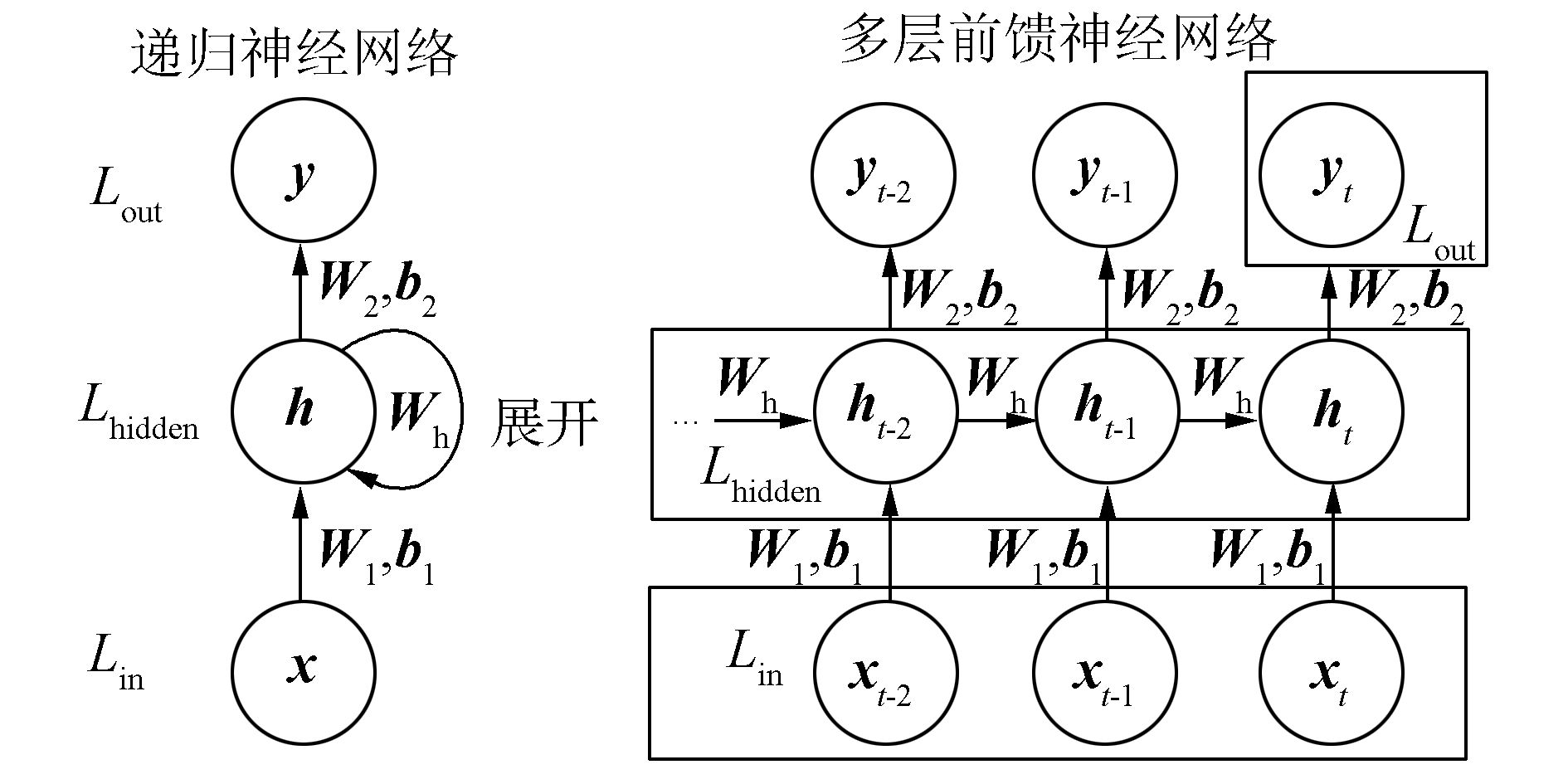图 3 递归神经网络展开过程 Fig.3 The unfolding of recurrent neural networks

1) 每个隐含层的输入都由两部分组成，即对应时刻输入层的输出和上一时刻隐含层的输出。而传统前馈神经网络的输入只能是上一层(隐含层或输入层)的输出。

2) 如图 3所示，展开的递归神经网络相同位置的参数W1b1W2b2Wh是共享的，而传统前馈神经网络则是非共享的，这也大大地降低了网络所要学习的参数。

3) 对于递归神经网络，每一个时刻都会给出一个输出，但是并不是必要的，只有t时刻的输出yt才是模型的预测结果，其余的输出结果都保存在了隐含层中。

1.3 递归神经网络用于风暴潮增水预测

 \begin{align} & \quad \quad \quad \quad \quad \quad \mathit{\boldsymbol{X}}=\left( {{\mathit{\boldsymbol{x}}}_{1}},{{\mathit{\boldsymbol{x}}}_{2}},\cdots ,{{\mathit{\boldsymbol{x}}}_{T-k}} \right)= \\ & \left\{ \left\{ {{d}_{1}},{{d}_{2}},\cdots ,{{d}_{~}}_{k} \right\},\left\{ {{d}_{2}},{{d}_{3}},\cdots ,{{d}_{~}}_{k+1} \right\},\cdots ,\left\{ {{d}_{T-k}},\cdots ,{{d}_{T-k+1}},\cdots ,{{d}_{T-1}} \right\} \right\} \\ & \quad \quad \mathit{\boldsymbol{y}}=\left\{ {{\mathit{\boldsymbol{y}}}_{1}},{{\mathit{\boldsymbol{y}}}_{2}},\cdots ,{{\mathit{\boldsymbol{y}}}_{T-k}} \right\}=\left\{ {{d}_{k+1}},{{d}_{k+2}},\cdots ,{{d}_{T}} \right\} \\ \end{align}

 $\begin{array}{l} \mathit{\boldsymbol{\hat X}} = \{ \left\{ {{\rm{ }}{\mathit{\boldsymbol{x}}_1},{\mathit{\boldsymbol{x}}_2}, \ldots ,{\mathit{\boldsymbol{x}}_l}} \right\},\left\{ {{\mathit{\boldsymbol{x}}_2},{\mathit{\boldsymbol{x}}_3}, \ldots ,{\mathit{\boldsymbol{x}}_{l + 1}}} \right\}, \ldots ,\\ \quad \quad \quad \{ {\mathit{\boldsymbol{x}}_{T - k - l + 1}},{\mathit{\boldsymbol{x}}_{T - k - l + 2}}, \ldots ,{\mathit{\boldsymbol{x}}_{T - k}}\} \} \\ \mathit{\boldsymbol{\hat y}} = \{ \left\{ {{\mathit{\boldsymbol{y}}_1},{\mathit{\boldsymbol{y}}_2}, \ldots ,{\mathit{\boldsymbol{y}}_l}} \right\},\left\{ {{\mathit{\boldsymbol{y}}_2},{\mathit{\boldsymbol{y}}_3}, \ldots ,{\mathit{\boldsymbol{y}}_{l + 1}}} \right\}, \ldots ,\\ \quad \quad \quad \left\{ {{\mathit{\boldsymbol{y}}_{T - k - l + 1}},{\mathit{\boldsymbol{y}}_{T - k - l + 2}}, \ldots ,{\mathit{\boldsymbol{y}}_{T - k}}} \right\}\} \end{array}$
2 实验结果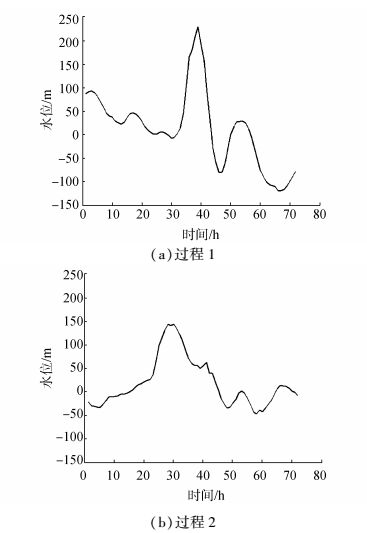图 4 风暴潮增水过程 Fig.4 The process of storm surge

 $\hat x = \frac{{x - {x_{{\rm{min}}}}}}{{{x_{{\rm{max}}}} - {x_{{\rm{min}}}}}}$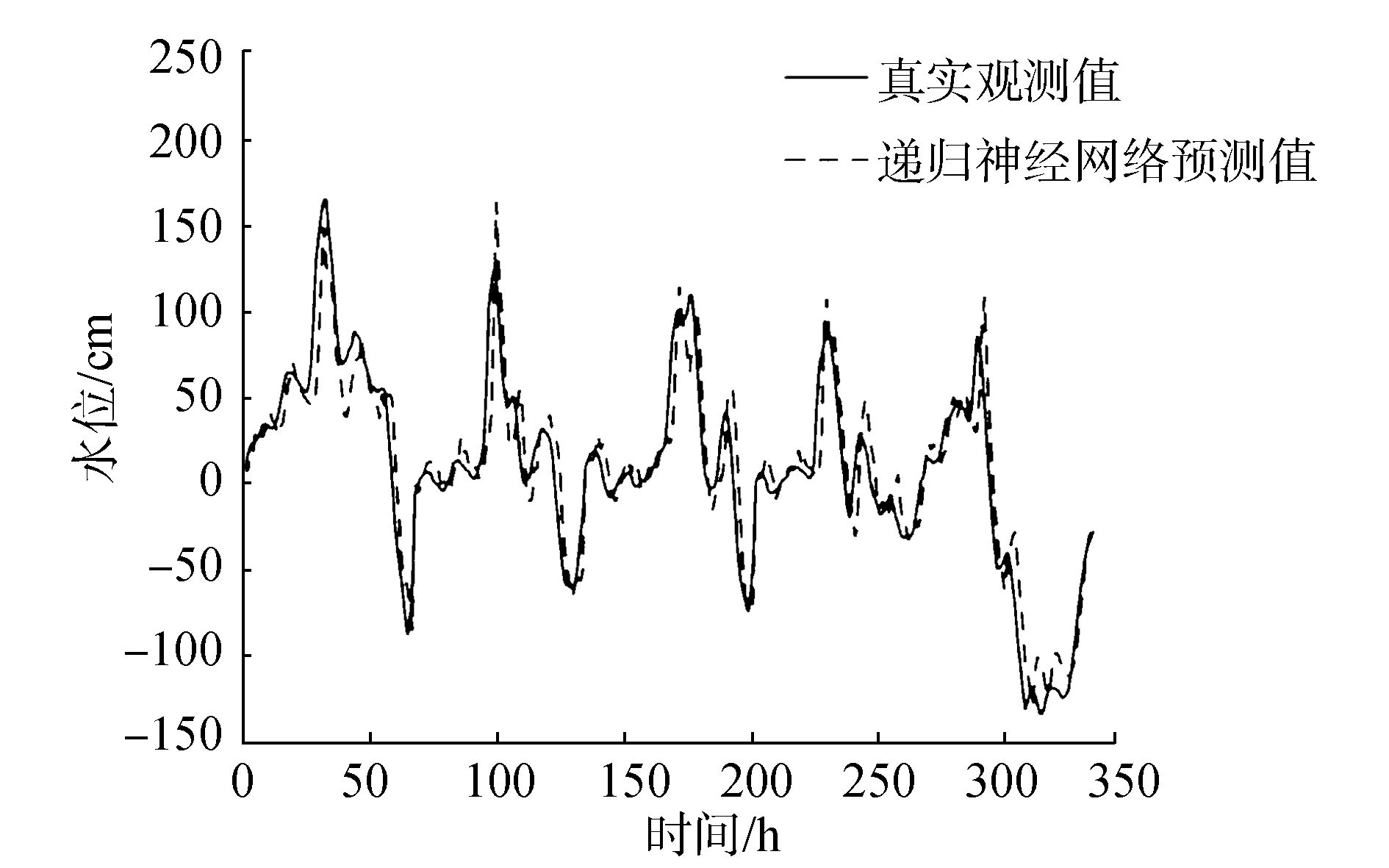图 5 递归神经网络3 h预测结果 Fig.5 The prediction of recurrent neural network for 3 hour later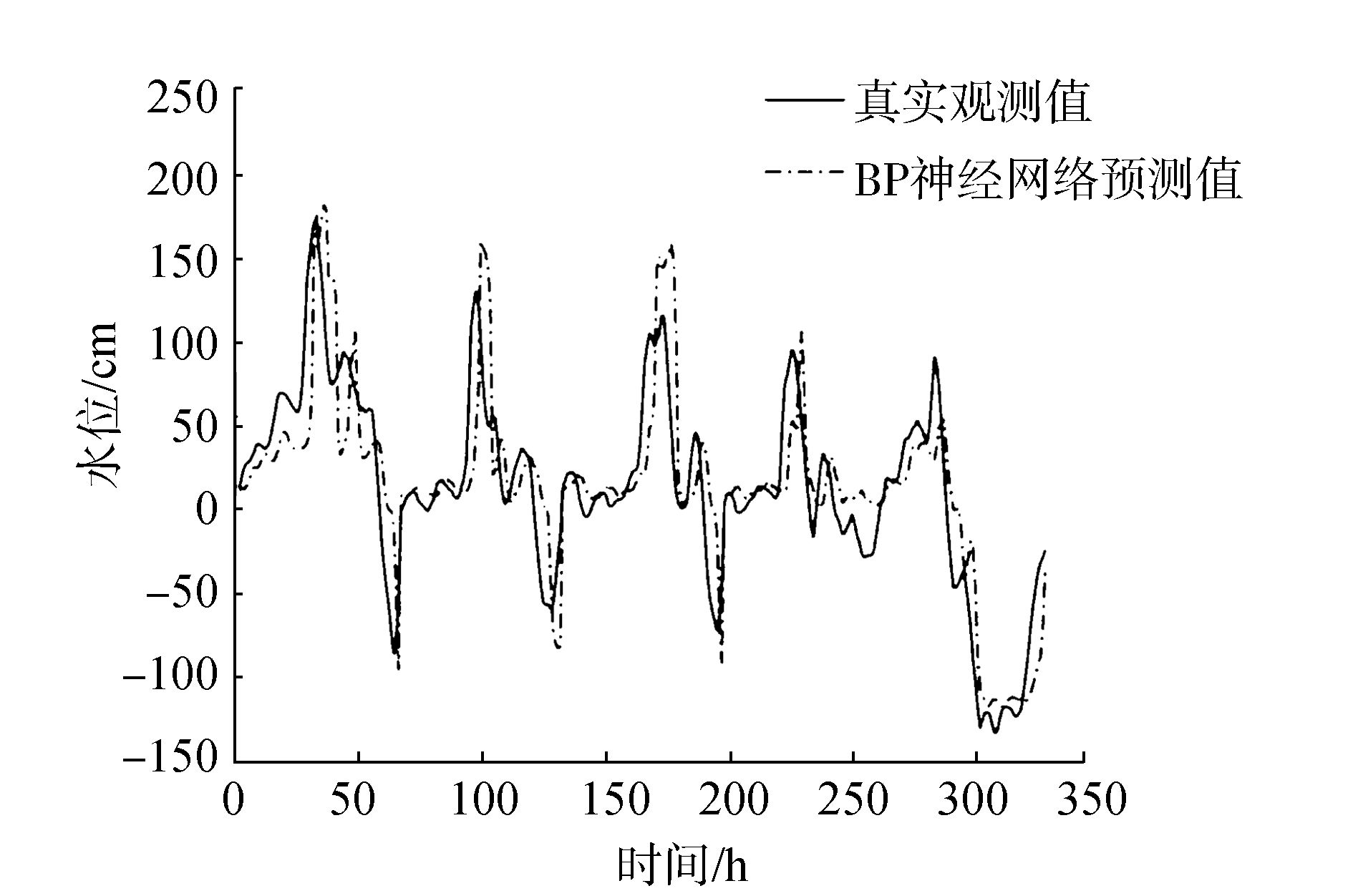图 6 BP神经网络3 h预测结果 Fig.6 The prediction of back propagation network (multilayer perceptron) for 3 hour later

 ${\rm{MAE = }}\frac{1}{N}\sum\limits_i^N {\left| {{y_i} - {{\hat y}_i}} \right|}$表 1 2014年增水过程预测的平均绝对误差 Tab.1 The mean absolute error of the prediction of storm surge in 2014
3 结束语

  王喜年. 风暴潮预报知识讲座[J]. 海洋预报, 2001, 18(1): 73-78. DOI:10.11737/j.issn.1003-0239.2001.01.010 (0)  高清清, 曹兵, 高鑫鑫, 等. 南通沿海台风风暴潮分析及其经验预报初探[J]. 海洋预报, 2014, 31(1): 29-35. GAO Qingqing, CAO Bing, GAO Xinxin, et al. Analysis of the typhoon storm surge in the Nantong coastal zone and the forecasting formulas[J]. Marine forecasts, 2014, 31(1): 29-35. (0)  曾德美. 青岛港风暴潮经验统计预报[J]. 海洋预报, 1992, 9(3): 66-73. ZENG Demei. A statistical forecasting of storm surge in qingdao harbor[J]. Marine forecasts, 1992, 9(3): 66-73. DOI:10.11737/j.issn.1003-0239.1992.03.009 (0)  谢亚力, 黄世昌. 钱塘江河口风暴潮经验预报[J]. 海洋预报, 2006, 23(1): 54-58. XIE Yali, HUANG Shichang. Effects on watercourse and hydrological factor in downstrean nanxi river due to water diversion[J]. Marine forecasts, 2006, 23(1): 54-58. DOI:10.11737/j.issn.1003-0239.2006.01.009 (0)  朱建荣, 朱首贤. ECOM模式的改进及在长江河口、杭州湾及邻近海区的应用[J]. 海洋与湖沼, 2003, 34(4): 364-373. ZHU Jianrong, ZHU Shouxian. Improvement of the ECOM with application to the changjiang river estuary, Hangzhou bay and adjacent waters[J]. Oceanologia Et limnologia sinica, 2003, 34(4): 364-373. DOI:10.11693/hyhz200304003003 (0)  黄世昌, 李玉成, 赵鑫, 等. 浙江沿海超强台风作用下风暴潮增水数值分析[J]. 海洋工程, 2008, 26(3): 58-64. HUANG Shichang, LI Yucheng, ZHAO Xin, et al. Numerical analysis of storm surge due to a super typhoon in coastal region of Zhejiang province[J]. The ocean engineering, 2008, 26(3): 58-64. (0)  王培涛, 于福江, 刘秋兴, 等. 福建沿海精细化台风风暴潮集合数值预报技术研究及应用[J]. 海洋预报, 2010, 27(5): 7-15. DOI:10.11737/j.issn.1003-0239.2010.05.002 (0)  LEE T L. Neural network prediction of a storm surge[J]. Ocean engineering, 2006, 33(3): 483-494. (0)  RAJASEKARAN S, GAYATHRI S, LEE T L, et al. Support vector regression methodology for storm surge predictions[J]. Ocean engineering, 2008, 35(16): 1578-1587. DOI:10.1016/j.oceaneng.2008.08.004 (0)  WILLIAMS R J, ZIPSER D. A learning algorithm for continually running fully recurrent neural networks[J]. Neural computation, 1989, 1(2): 270-280. DOI:10.1162/neco.1989.1.2.270 (0)  ELMAN J L. Finding structure in time[J]. Cognitive science, 1990, 14(2): 179-211. DOI:10.1207/s15516709cog1402_1 (0)  BOTVINICK M M, PLAUT D C. Short-term memory for serial order:a recurrent neural network model[J]. Psychological review, 2006, 113(2): 201-233. DOI:10.1037/0033-295X.113.2.201 (0)  SAINATH T N, KINGSBURY B, SAON G, et al. Deep convolutional neural networks for large-scale speech tasks[J]. Neural networks, 2015, 64: 39-48. DOI:10.1016/j.neunet.2014.08.005 (0)  SARIKAYA R, HINTON G E, DEORAS A, et al. Application of deep belief networks for natural language understanding[J]. IEEE transactions on audio, speech, and language processing, 2014, 22(4): 778-784. DOI:10.1109/TASLP.2014.2303296 (0)  KRIZHEVSKY A, SUTSKEVER I, HINTON G E, et al. ImageNet classification with deep convolutional neural networks[C]//International Conference on Neural Information Processing Systems. Nevada, America, 2012:1097-1105. (0)  SVOZIL D, KVASNICKA V, POSPICHAL J, et al. Introduction to multi-layer feed-forward neural networks[J]. Chemometrics and intelligent laboratory systems, 1997, 39(1): 43-62. DOI:10.1016/S0169-7439(97)00061-0 (0)  HORNIK K, STINCHCOMBE M B, WHITE H, et al. Multilayer feedforward networks are universal approximators[J]. Neural networks, 1989, 2(5): 359-366. DOI:10.1016/0893-6080(89)90020-8 (0)  RUMELHART D E, HINTON G E, WILLIAMS R J, et al. Learning representations by back-propagating errors[J]. Nature, 1988, 323(6088): 533-536. (0)  KOSMATOPOULOS E B, POLYCARPOU M M, CHRISTODOULOU M A, et al. High-order neural network structures for identification of dynamical systems[J]. IEEE transactions on neural networks, 1995, 6(2): 422-431. DOI:10.1109/72.363477 (0)  WERBOS P J. Backpropagation through time:what it does and how to do it[J]. Proceedings of the IEEE, 1990, 78(10): 1550-1560. DOI:10.1109/5.58337 (0)